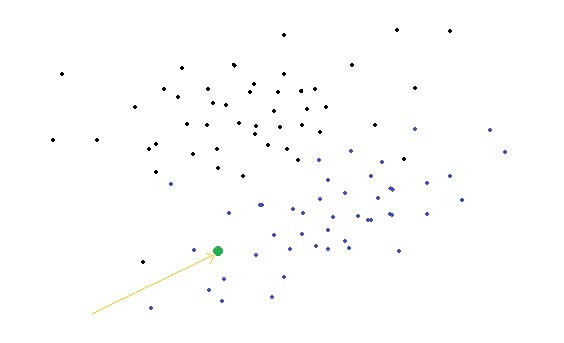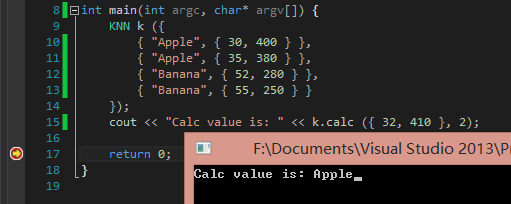# C++机器学习（1）k-近邻算法

```1 mov eax, 10h```

```1 2 3 4 5 push ebx mov ebx, 12h sub ebx, 2h mov eax, ebx pop ebx```

```1 int i = 16;```

```1 int i=18-2;``````1 2 3 4 5 6 7 8 9 10 11 12 13   //#ifdef UNICODE //typedef std::string string_t; //#else //typedef std::wstring string_t; //#endif   //下面用于计算两个双精度浮点类型数据是否相等 bool double_is_equal (double a, double b) { static const double double_error = 0.000001; if (a == 0 || b == 0) return fabs (a + b) < double_error; return fabs (fabs (a) < fabs (b) ? ((b - a) / a) : ((a - b) / b)) < double_error; }```

```1 2 3 4 5 6 7 8 9 10 11 12 13 14 15 16 17 18 19 20 21 22 23 24 25 //特征类，一个类对象代表单个数据的特征集 class Feature_Data { public: Feature_Data (std::initializer_list<double> dat) { data.assign (dat.begin (), dat.end ()); }   Feature_Data (std::vector<double> dat) { data.swap (dat); }   //获取特征数量 ptrdiff_t get_size () { return data.size (); }   //重载[]运算符，后面将会以奇怪的方式访问数据 double operator[](ptrdiff_t index) { return data [index]; }   private: //特征集 std::vector<double> data; };```

```1 2 3 4 5 6 7 8 9 10 11 12 13 14 15 //数据类，一个类对象代表一个特征集与一个标签 class Feature_Object : public Feature_Data { public: Feature_Object (std::string _label, std::initializer_list<double> dat) : Feature_Data (dat) { label = _label; }   //如果想知道特征数据所对应的品牌，那么就调用这函数咯 std::string get_label () { return label; }   private: std::string label; };```

```1 2 3 4 5 6 7 8 9 10 11 12 13 14 15 16 17 18 19 20 21 22 23 24 25 26 27 //数据组类，一个类对象代表一组数据类类对象 class Feature_Object_Collection { public: //这个主要是为了方便构建测试数据而弄的，比较方便 Feature_Object_Collection (std::initializer_list<Feature_Object> list) { data.assign (list.begin (), list.end ()); }   Feature_Object_Collection (const Feature_Object_Collection &o) { data.assign (o.data.begin (), o.data.end ()); }   //... //(○´･д･)ﾉ 可恶的博主，到底省略了什么代码？？   ptrdiff_t get_size () { return data.size (); }   //又是以奇怪的方式访问数据 Feature_Object& operator[](ptrdiff_t index) { return data [index]; }   protected: std::vector<Feature_Object> data; };```

```1 2 3 4 5 6 7 8 9 10 11 12 13 14 15 16 17 18 19 20 21 22 23 24 25 26 27 28 29 30 31 32 33 34 35 36 37 38 39 40 41 42 43 44 45 46 47 48 49 50 51 52 53 54 55 56 57 58 59 60 61 62 63 64 65 //K-近邻算法实现 class KNN : public Feature_Object_Collection { public: KNN (std::initializer_list<Feature_Object> list) : Feature_Object_Collection (list) {}   KNN (Feature_Object_Collection& o) : Feature_Object_Collection (o) {}   KNN (const KNN &o) : Feature_Object_Collection (o) {}   std::string calc (Feature_Data dat, ptrdiff_t k) { //k-近邻算法含义是，从给定数据集中找出与目标数据最近的k个数据集 //如果标签都一样，那么就返回标签。如果标签不同，那么计算后给出 //标签出现次数最多的标签。由于只保留距离最近的k个数据，所以... pFeature_Object *o = new pFeature_Object [k]; double *sqc = new double [k];   ptrdiff_t i, j, used, count = data.size (), size = dat.get_size (); double squared = 0; 遍历所有特征 for (i = used = 0; i < count; ++i, squared = 0) { //求方差 for (j = 0; j < size; ++j) squared += (pow (data [i] [j] - dat [j], 2)); squared = ::pow (squared, 0.5);   if (used < k) { //好险，数据还不够k个，可以安心插入 ++used; j = used - 1; } else if (sqc [k - 1] <= squared) { //哦豁，方差比已有的k个数据中方差最大的还大，只能做个无辜的路人 j = -1; } else { j = used - 1; } //在插入数据时为了不把大小顺序弄乱，所以遍历一下，找到最佳插入点 for (; j > 0; o [j] = o [j - 1], sqc [j] = sqc [j - 1], --j) { if (sqc [j - 1] < squared) { sqc [j] = squared; o [j] = &data [i]; break; } } //我去，方差够小哇，可以排第零了 if (j == 0) { o  = &data [i]; sqc  = squared; } }   //下面已经有了used个数据了，那么就全部塞入map里面吧，找到出现次数最多的标签 std::map<std::string, ptrdiff_t> m; std::string s; for (i = 0; i < used; ++i) ++m [o [i]->get_label ()]; for (i = 0; i < used; ++i) { if (i == 0 || j < m [o [i]->get_label ()]) { j = m [s = o [i]->get_label ()]; } }   delete sqc; delete o; return s; } };```

```1 2 3 4 5 6 7 8 9 10 11 int main(int argc, char* argv[]) { KNN k ({ { "Apple", { 30, 400 } }, { "Apple", { 35, 380 } }, { "Banana", { 52, 280 } }, { "Banana", { 55, 250 } } }); cout << "Calc value is: " << k.calc ({ 32, 410 }, 2);   return 0; }```